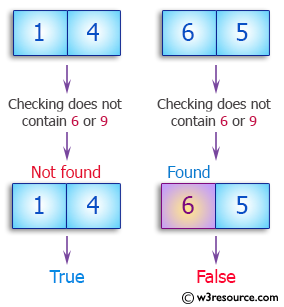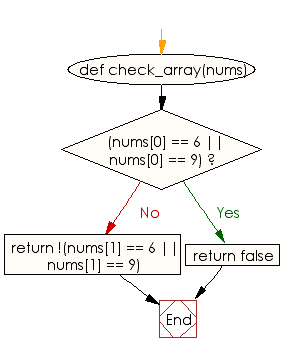﻿ Ruby Array exercises: Check whether an given array of integers of length 2 does not contain a 6 or a 9 - w3resource# Ruby Array Exercises: Check whether an given array of integers of length 2 does not contain a 6 or a 9

## Ruby Array: Exercise-18 with Solution

Write a Ruby program to check whether an given array of integers of length 2 does not contain a 6 or a 9.Ruby Code:

``````def check_array(nums)
if(nums == 6 || nums == 9)
return false
end
return !(nums == 6 || nums == 9)
end

print check_array([1, 4]),"\n"
print check_array([6, 5]),"\n"
print check_array([5, 9])
``````

Output:

```true
false
false
```

Flowchart:Ruby Code Editor: